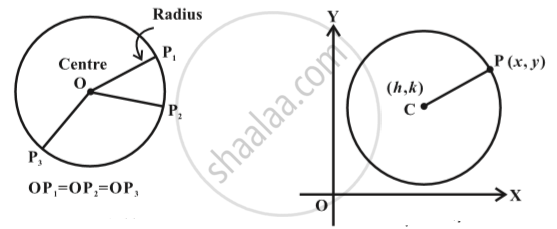Share

# Concept of Circle

#### definition

A circle is the set of all points in a plane that are equidistant from a fixed point in the plane.

#### notes

The fixed point is called the centre of the circle and the distance from the centre to a point on the circle is called the radius of the circle Fig.The equation of the circle is simplest if the centre of the circle is at the origin. However, we derive below the equation of the circle with a given centre and radius in above figure.
Given C (h, k) be the centre and r the radius of circle. Let P(x, y) be any point on the circle in the above figure.
By the distance formula, we have  sqrt((x-h)^2 + (y-k)^2)=r

i.e. (x-h)^2 + (y-k)^2 =r^2

### Shaalaa.com

Circle [00:28:17]
S
0%

S